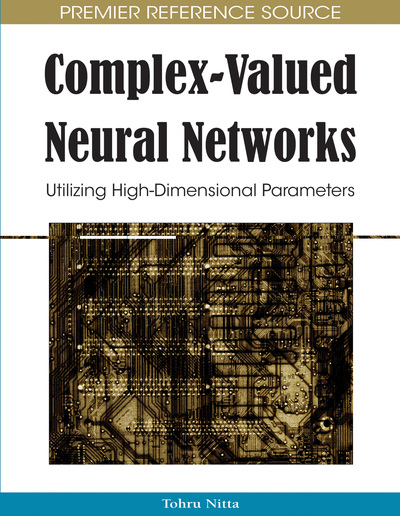# Global Stability Analysis for Complex-Valued Recurrent Neural Networks and Its Application to Convex Optimization Problems

Mitsuo Yoshida, Takehiro Mori
DOI: 10.4018/978-1-60566-214-5.ch005
OnDemand:
(Individual Chapters)
Available
\$37.50
No Current Special Offers

## Abstract

Global stability analysis for complex-valued artificial recurrent neural networks seems to be one of yet-unchallenged topics in information science. This chapter presents global stability conditions for discrete-time and continuous- time complex-valued recurrent neural networks, which are regarded as nonlinear dynamical systems. Global asymptotic stability conditions for these networks are derived by way of suitable choices of activation functions. According to these stability conditions, there are classes of discrete-time and continuous-time complex-valued recurrent neural networks whose equilibrium point is globally asymptotically stable. Furthermore, the conditions are shown to be successfully applicable to solving convex programming problems, for which real field solution methods are generally tedious.
Chapter Preview
Top

## Introduction

Recurrent neural networks whose neurons are fully interconnected have been utilized to implement associative memories and solve optimization problems. These networks are regarded as nonlinear dynamical feedback systems. Stability properties of this class of dynamical networks are an important issue from applications point of view.

On the other hand, several models of neural networks that can deal with complex numbers, the complex-valued neural networks, have come to forth in recent years. These networks have states, connection weights, and activation functions, which are all complex-valued. Such networks have been studied in terms of their abilities of information processing, because they possess attractive features which do not exist in their real-valued counterparts (Hirose, 2003; Kuroe, Hashimoto & Mori, 2001, 2002; Kuroe, Yoshida & Mori, 2003; Nitta, 2000; Takeda & Kishigami, 1992; Yoshida, Mori & Kuroe, 2004; Yoshida & Mori, 2007). Generally, activation functions of neural networks crucially determine their dynamic behavior. In complex-valued neural networks, there is a greater choice of activation functions compared to real-valued networks. However, the question of appropriate activation functions has been paid insufficient attention to in the past.

Local asymptotic stability conditions for complex-valued recurrent neural networks with an energy function defined on the complex domain have been studied earlier and synthesis of complex-valued associative memories has been realized (Kuroe et al., 2001, 2002). However, studies on their application to global optimization problems and theoretical analysis for global asymptotic stability conditions remain yet-unchallenged topics.

The purpose of this chapter is to analyze global asymptotic stability for complex-valued recurrent neural networks. Two types of complex-valued recurrent neural networks are considered: discrete-time model and continuous-time model. We present global asymptotic stability conditions for both models of the complex-valued recurrent neural networks. To ensure global stability, classes of complex-valued functions are defined as the activation functions, and therewith several stability conditions are obtained. According to these conditions, there are classes of discrete-time and continuous-time complex-valued recurrent neural networks whose common equilibrium point is globally asymptotically stable. Furthermore, the obtained conditions are shown to be successfully applicable to solving convex programming problems.

The chapter is organized as follows. In Background, a brief summary of applications to associative memories and optimization problems in real-valued recurrent neural networks are presented. Moreover, results on stability analysis and applications of these real-valued neural networks are introduced. Next, models of discrete-time and continuous-time complex-valued neural networks are described. For activation functions of these networks, two classes of complex-valued function are defined. In the next section, global asymptotic stability conditions for the discrete-time and continuous-time complex-valued neural networks are proved, respectively. Some discussions thereof are also given. Furthermore, applications of complex-valued neural networks to convex programming problems with numerical examples are shown in the subsequent section. Finally, concluding remarks and future research directions are given.

## Complete Chapter List

Search this Book:
Reset